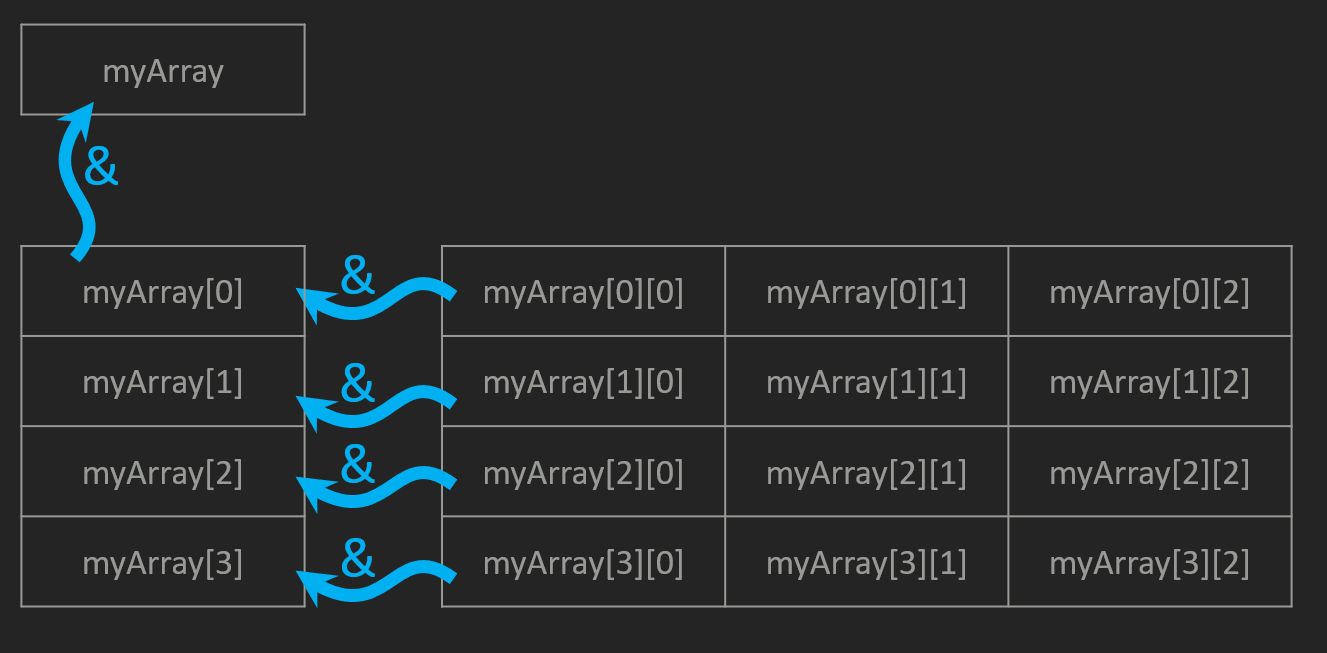An array in C/C++ is an object, like a class. Associated with the variable name is relative information: data type (double, int, char), the size of each element, the number of elements, etc. To define an array object:

`int myArray;`

This definition will allocate memory for five integers placed consecutivly. Similarly, multidimensional arrays can be defined using this syntax:

`int my2dArray;`

Here, 50 consecutive bytes of memory are allocated. The elements are then accessed or assigned by:

```myArray = 20;               //set 4rd element to a value of 20
my2DArray = 15;          //set 4rd row, 2nd column to 15
myArray = my2dArray;  //set 1st element equal to first row, first column
```

Array objects can also be passed as arguments to functions:

```double addElements(double arrayToAdd) {
int sum = 0;
for (int i = 0; i < numElements; i++) {
}
return sum;
}

double myArray = { 1,2,3,4 };
```

When array objects themselves are passed as functions, the compiler will be able to track the relative information and enable the use of utilities such as the sizeof() function.
The caveat: While array objects are efficient, the size of the array must be known at the time the .exe is compiled. Another complexity that is tricky for the beginner is that array objects are scope limited, that means there elements can only be access inside function blocks in which they were declared.

When the size of an array cannot be known at the time of compilation, or where it is desired to have a less limited scope, C and C++ enable the use of dynamic memory (also search stack vs., heap). This memory space is slightly less efficient but the size of objects can be defined while the program is running. Additionally, the memory management is wholly left up to the developer!
When dynamic memory is used, arrays are referenced using a pointer that points to the first element in the array. In C++ the new keyword returns a pointer to the newly allocated array in dynamic memory. Note: for every place the new keyword is used an associated delete keyword must be used (search memory leaks). A dynamic array of integers would be allocated and accessed as such:

```int* array2 = NULL;					//create a pointer to be used to reference integer array
//allocate memory on the heap for an array of 100 integers
array2 = new int;				//the bit values will all be zero by default (0 for unsigned int, -(2^n-1) for signed)
array2 = 55;						//set the value of the third element to 55
//get the size in bytes of an integer
//multiply by 5 and add the result to the address of the first element of array2
*(array2 + 5 * sizeof(int)) = 67;		//dereference the resulting address and set its value to 67 (5th element)
delete[] array2;					//delete contents of heap - does not delete pointer
array2 = NULL;					//set pointer value to NULL
```

If we think more abstractly, we can create a pointer to any data type and then create an array of those elements using the new keyword. For example we can create an array of pointers:

```int* oneD_array = NULL;     //create a pointer (to an int) to store locaton of new array
oneD_array = new int;     //new returns pointer to first element of dynamic memory
delete[] oneD_array;	     //delete single array of integers

int** twoD_array = NULL;     //create a pointer (to a pointer to an int)
twoD_array = new int*;   //use new keyword to allocate a single array of pointers
delete[] twoD_array;              //delete single array of pointers
```

Notice the array that we created does not actually contain data that represents a 2D array, it is a single array of pointers. But we can then use the new keyword to return a memory location for each pointer element in the array that does point to an allocated integer array. Notice that this does not create a contiguous block of data, but in very large file formats, this may be favorable to not have to defrag the memory.

```int** myArray2 = new int*;	//allocate array of pointers on heap
for (int i = 0; i < 50; i++) {
myArray2[i] = new int;
}
myArray2 = 15;			//set value
for (int i = 0; i < 50; i++) {		//delete each int array
delete[] myArray2[i];
myArray2[i] = NULL;
}
delete[] myArray2;				//delete array of pointers
myArray2 = NULL;				//set myArray to NULL
```The delete[] function only deletes the memory. After the function returns, the value is retained in the pointer. To avoid atempting to dereference memory which is now invalid, it is a good habit to set the value of the pointer to NULL.

Because each dimension beyond the first is just a list of references, there is no geometric equivalent and therefore is not limited a finite number of dimensions.